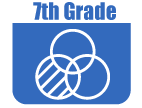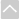OER Curriculum

These Open Educational Resources are comprehensive and coherent curricular materials that may be used to teach a course or grade level.

Utah Middle School Project
• Chapter 1 - Mathematical Foundation (UMSMP)
This is Chapter 1 of the Utah Middle School Math: Grade 7 textbook. It provides a Mathematical Foundation for Probability, Percent, Rational Number Equivalence.
• Chapter 1 - Student Workbook (UMSMP)
This is Chapter 1 of the Utah Middle School Math: Grade 7 student workbook. It covers the following topics: Probability, Percent, Rational Number Equivalence.
• Chapter 2 - Mathematical Foundation (UMSMP)
This is Chapter 2 of the Utah Middle School Math: Grade 7 textbook. It provides a Mathematical Foundation for Rational Number Operations.
• Chapter 2 - Student Workbook (UMSMP)
This is Chapter 2 of the Utah Middle School Math: Grade 7 student workbook. It covers Rational Number Operations.
• Chapter 3 - Mathematical Foundation (UMSMP)
This is Chapter 3 of the Utah Middle School Math: Grade 7 textbook. It provides a Mathematical Foundation for Expressions and Equations I.
• Chapter 3 - Student Workbook (UMSMP)
This is Chapter 3 of the Utah Middle School Math: Grade 7 student workbook. It covers Expressions and Equations I.
• Chapter 4 - Mathematical Foundation (UMSMP)
This is Chapter 4 of the Utah Middle School Math: Grade 7 textbook. It provides a Mathematical Foundation for Proportional Relationships and Solving Problems.
• Chapter 4 - Student Workbook (UMSMP)
This is Chapter 4 of the Utah Middle School Math: Grade 7 student workbook. It focuses on these topics: Proportional Relationships and Solving Problems.
• Chapter 5 - Mathematical Foundation (UMSMP)
This is Chapter 5 of the Utah Middle School Math: Grade 7 textbook. It provides a Mathematical Foundation for Geometry I - Scale Drawings, Geometric Figures.
• Chapter 5 - Student Workbook (UMSMP)
This is Chapter 5 of the Utah Middle School Math: Grade 7 student workbook. It focuses on these topics: Geometry I - Scale Drawings and Geometric Figures.
• Chapter 6 - Mathematical Foundation (UMSMP)
This is Chapter 6 of the Utah Middle School Math: Grade 7 textbook. It provides a Mathematical Foundation for Expressions and Equations II - Real-world equations, Inequalities.
• Chapter 6 - Student Workbook (UMSMP)
This is Chapter 6 of the Utah Middle School Math: Grade 7 textbook. It focuses on these topics: Real-world equations and Inequalities.
• Chapter 7 - Mathematical Foundation (UMSMP)
This is Chapter 7 of the Utah Middle School Math: Grade 7 textbook. It provides a Mathematical Foundation for Expressions and Equations II - Real-world equations, Inequalities.
• Chapter 7 - Student Workbook (UMSMP)
This is Chapter 7 of the Utah Middle School Math: Grade 7 student workbook. It focuses on Probability and Statistics.
• Chapter 8 - Mathematical Foundation (UMSMP)
This is Chapter 8 of the Utah Middle School Math Grade 7 textbook. It provides a Mathematical Foundation for Measurement in 2-3 Dimensions and Cross-Sections of Solids.
• Chapter 8 - Student Workbook (UMSMP)
This is Chapter 8 of the Utah Middle School Math Grade 7 student workbook. It focuses on Measurement in 2-3 Dimensions and Cross-Sections of Solids.
•Engage NY
• Grade 7 Math Module 1:Ratios and Proportional Relationship (EngageNY)
In this 30-day Grade 7 module, students build upon sixth grade reasoning of ratios and rates to formally define proportional relationships and the constant of proportionality. Students explore multiple representations of proportional relationships by looking at tables, graphs, equations, and verbal descriptions. Students extend their understanding about ratios and proportional relationships to compute unit rates for ratios and rates specified by rational numbers. The module concludes with students applying proportional reasoning to identify scale factor and create a scale drawing.
• Grade 7 Math Module 2: Rational Numbers (EngageNY)
In Grade 6, students formed a conceptual understanding of integers through the use of the number line, absolute value, and opposites and extended their understanding to include the ordering and comparing of rational numbers.This module uses the Integer Game: a card game that creates a conceptual understanding of integer operations and serves as a powerful mental model students can rely on during the module. Students build on their understanding of rational numbers to add, subtract, multiply, and divide signed numbers. Previous work in computing the sums, differences, products, and quotients of fractions serves as a significant foundation.
• Grade 7 Math Module 3: Expressions and Equations (EngageNY)
This module consolidates and expands upon students understanding of equivalent expressions as they apply the properties of operations to write expressions in both standard form and in factored form. They use linear equations to solve unknown angle problems and other problems presented within context to understand that solving algebraic equations is all about the numbers. Students use the number line to understand the properties of inequality and recognize when to preserve the inequality and when to reverse the inequality when solving problems leading to inequalities. They interpret solutions within the context of problems. Students extend their sixth-grade study of geometric figures and the relationships between them as they apply their work with expressions and equations to solve problems involving area of a circle and composite area in the plane, as well as volume and surface area of right prisms.
• Grade 7 Math Module 4: Percent and Proportional Relationships (EngageNY)
In Module 4, students deepen their understanding of ratios and proportional relationships from Module 1 by solving a variety of percent problems. They convert between fractions, decimals, and percents to further develop a conceptual understanding of percent and use algebraic expressions and equations to solve multi-step percent problems. An initial focus on relating 100% to the whole serves as a foundation for students. Students begin the module by solving problems without using a calculator to develop an understanding of the reasoning underlying the calculations.
• Grade 7 Math Module 5: Statistics and Probability (EngageNY)
In this module, students begin their study of probability, learning how to interpret probabilities and how to compute probabilities in simple settings. They also learn how to estimate probabilities empirically. Probability provides a foundation for the inferential reasoning developed in the second half of this module. Additionally, students build on their knowledge of data distributions that they studied in Grade 6, compare data distributions of two or more populations, and are introduced to the idea of drawing informal inferences based on data from random samples.
• Grade 7 Math Module 6: Geometry (EngageNY)
In Module 6, students delve further into several geometry topics they have been developing over the years. Grade 7 presents some of these topics, (e.g., angles, area, surface area, and volume) in the most challenging form students have experienced yet. Module 6 assumes students understand the basics. The goal is to build a fluency in these difficult problems. The remaining topics, (i.e., working on constructing triangles and taking slices (or cross-sections) of three-dimensional figures) are new to students.
•Georgia Standards of Excellence Curriculum Frameworks
• Grade 7 Unit 1: Operations with Rational Numbers (Georgia Standards)
The units in this instructional framework emphasize key standards that assist students to develop a deeper understanding of numbers. They learn to express different representations of rational numbers (e.g., fractions, decimals, and percents) and interpret negative numbers in everyday context (e.g., sea level change). The big ideas that are expressed in this unit are integrated with such previous knowledge as estimation, mental and basic computation. All of these concepts need to be reviewed throughout the year.
• Grade 7 Unit 2: Expressions and Equations (Georgia Standards)
The units in this instructional framework emphasize key standards that assist students to develop a deeper understanding of numbers. They learn how to solve multi- step equations and discuss the difference between equations and expressions. The Big Ideas that are expressed in this unit are integrated with such routine topics as estimation, mental and basic computation. All of these concepts need to be reviewed throughout the year.
• Grade 7 Unit 3: Ratio and Proportional Relationships (Georgia Standards)
The units in this instructional framework emphasize key standards that assist students to develop a deeper understanding of numbers. They learn to express different representations of rational numbers (e.g., fractions, decimals, and percents), discover how to identify and explain the constant of proportionality, and represent proportional relationships and scale drawings within real-world contexts. The Big Ideas that are expressed in this unit are integrated with such routine topics as estimation, mental and basic computation. All of these concepts need to be reviewed throughout the year.
• Grade 7 Unit 4: Geometry (Georgia Standards)
The units in this instructional framework emphasize key standards that assist students to develop a deeper understanding of numbers. In this unit they will be engaged in using what they have previously learned about drawing geometric figures using rulers and protractor with an emphasis on triangles, students will also write and solve equations involving angle relationships, area, volume, and surface area of fundamental solid figures.
• Grade 7 Unit 5: Inferences (Georgia Standards)
The units in this instructional framework emphasize key standards that assist students in developing a deeper understanding of numbers. They have learned to recognize and express different representations of rational numbers. Now they will learn how to apply the statistical aspect of mathematics to the measures of center, variability and population. The Big Ideas that are expressed in this unit are integrated with such routine topics as estimation, mental and basic computation. All of these concepts need to be reviewed throughout the year.
• Grade 7 Unit 6: Statistics (Georgia Standards)
In this unit students will: Analyze data from many different sources such as organized lists, box-plots, bar graphs, histograms and dot plots. Understand that responses to statistical questions may vary. Understand that data can be described by a single number. Determine quantitative measures of center (median and/or mean). Determine quantitative measures of variability (interquartile range and range).
•The Online Core Resource pages are a collaborative project between the Utah State Board of Education and the Utah Education Network. If you would like to recommend a high quality resource, contact Trish French (Elementary) or Lindsey Henderson (Secondary). If you find inaccuracies or broken links contact resources@uen.org.